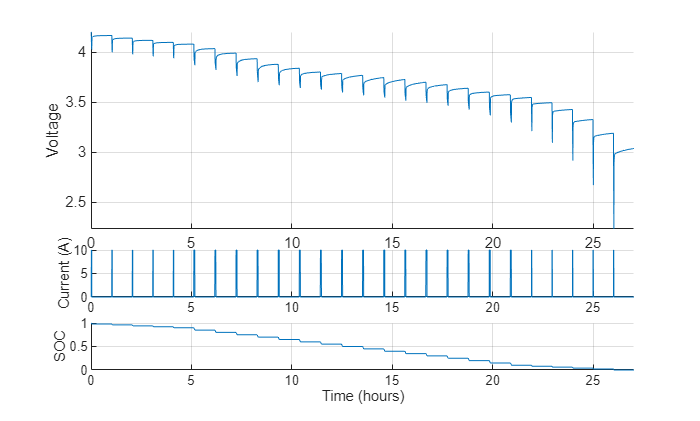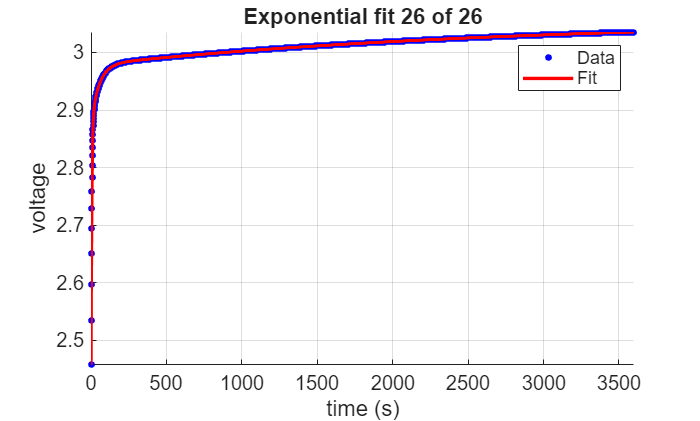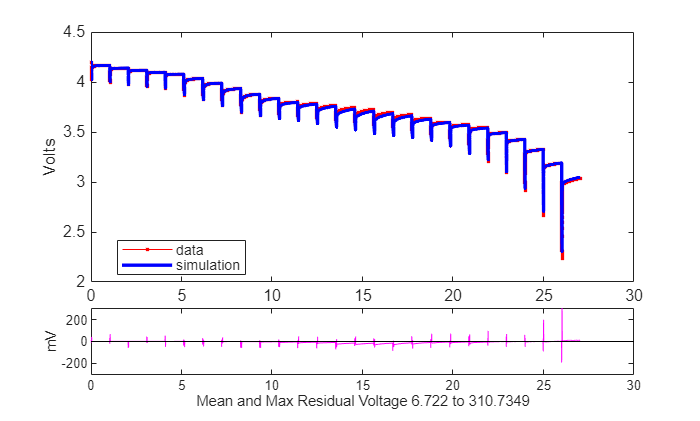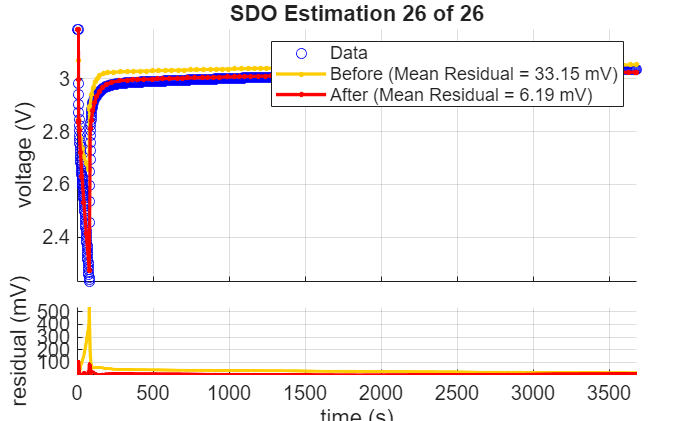## Generate Parameter Data for Equivalent Circuit Battery Block

Using MathWorks® tools, estimation techniques, and measured lithium-ion or lead acid battery data, you can generate parameters for the Equivalent Circuit Battery block. The Equivalent Circuit Battery block implements a resistor-capacitor (RC) circuit battery with open circuit voltage, series resistance, and 1 through N RC pairs. The number of RC pairs reflects the number of time constants that characterize the battery transients. Typically, the number of RC pairs ranges from 1 through 5.

To create parameter data for the Equivalent Circuit Battery block, follow these workflow steps. The steps use numerical optimization techniques to determine the number of recommended RC pairs, provide initial estimates for the battery model circuit parameters, and estimate parameters to fit a model to experimental pulse discharge data. The results provide the open circuit voltage, series resistance, and RC pair parameter data for the Equivalent Circuit Battery block.

WorkflowDescription

Estimate Equivalent Circuit Lithium-Ion Battery Data

Live script providing workflow steps 1 through 3.

Step 1: Load and Preprocess Data

Load and preprocess time series battery discharge voltage and current data.

Step 2: Determine the Number of RC Pairs

Determine the number of necessary time constants (TC) for estimation.

Step 3: Estimate Parameters

For battery discharge data, estimate and optimize:

• Open-circuit voltage, Em

• Series resistance, R0

• RC pair(s) time constant(s), Tau

• RC pair(s) resistance(s), Rx

Step 4: Set Equivalent Circuit Battery Block Parameters

Set the Equivalent Circuit Battery block parameters.

### Estimate Equivalent Circuit Lithium-Ion Battery Data

The workflow steps estimate data for an equivalent circuit lithium-ion polymer (LiPo) battery. The steps use numerical optimization techniques to determine the number of recommended RC pairs, provide initial estimates for the battery model circuit parameters, and estimate parameters to fit a model to experimental pulse discharge data. The results provide the open circuit voltage, series resistance, and RC pair parameter data.

Step 1: Load and Preprocess Data

Data Format and Requirements

The workflow supports pulse discharge sequences from 100% to 0% state-of-charge (SOC). The data requirements include:

• Time series consisting of current and voltage from an experimental pulse discharge. For each experimental data set, the temperature is constant. The sample rate should be a minimum of 1 Hz, with an ideal rate at 10 Hz.

• Voltage accuracy within ±5 mV, ideally within ±1 mV.

• Current accuracy within ±100 mA, ideally within 10 mA.

• Temperature accuracy within ±1 °C, ideally within ±1 °C.

• Change in SOC for each pulse should not be greater than 5%.

• Data collection at high or low SOC might need modification to ensure safety.

• Sufficient relaxation time after each pulse to ensure battery approaches steady-state voltage.

Load and preprocess time series discharge voltage and current data for a lithium-ion polymer (LiPo) battery.

First, create a `PulseSequence` object.

```psObj = Battery.PulseSequence; disp(psObj);```
``` PulseSequence with properties: Data: [0×5 double] ModelName: '' MetaData: [1×1 Battery.MetaData] Capacity: 1 CapacityAh: 2.7778e-04 NumPulses: 0 TestType: 'none' Time: [0×1 double] Voltage: [0×1 double] Current: [0×1 double] Charge: [0×1 double] ChargeAh: [0×1 double] SOC: [0×1 double] Parameters: [1×0 Battery.Parameters] ParametersHistory: [1×0 Battery.Parameters] idxEdge: [] idxLoad: [] idxRelax: [] Pulse: [0×1 Battery.Pulse] ```

Specify the file name and load the raw data.

```FileName = 'Synthetic_LiPo_PulseDischarge.mat'; [time,voltage,current] = Battery.loadDataFromMatFile(FileName);```

Add the data to the `PulseSequence`.

`psObj.addData(time,voltage,current);`

Review the pulse sequence data.

`psObj.plot();`Break up the data into `Battery.Pulse` objects by creating the objects within the `PulseSequence`. This creates a parameter object with look-up tables that are the correct size.

```psObj.createPulses(... 'CurrentOnThreshold',0.1,... %minimum current magnitude to identify pulse events 'NumRCBranches',3,... %how many RC pairs in the model 'RCBranchesUse2TimeConstants',false,... %do RC pairs have different time constant for discharge and rest? 'PreBufferSamples',10,... %how many samples to include before the current pulse starts 'PostBufferSamples',15); %how many samples to include after the next pulse starts```

Specify the model that matches the number of RC branches and time constants.

`psObj.ModelName = 'BatteryEstim3RC_PTBS';`

Plot the identified pulses in the data.

`psObj.plotIdentifiedPulses();`To exclude pulses, use this code.

```% psObj.removePulses(indexToRemove); % psObj.plotIdentifiedPulses();```

Step 2: Determine the Number of RC Pairs

Determine the number of RC pairs. Too many pairs can over-fit the data. Too few pairs increases the fit error. If you decide to change the number of pairs, you rerun `createPulses` and change the `NumRCBranches` to the new value.

Determine the number of necessary time constants (TC) for estimation.

```PulsesToTest = [1 floor(psObj.NumPulses/2), psObj.NumPulses-1]; psObj.Pulse(PulsesToTest).compareRelaxationTau();```Step 3: Estimate Parameters

Estimate the parameters. First, set parameter values. Only update parameters once. Updating the parameters changes the history.

```Params = psObj.Parameters; Params.Tx(1,:,:) = 5; Params.Tx(2,:,:) = 50; Params.Tx(3,:,:) = 200; Params.TxMin(1,:,:) = 1; Params.TxMax(1,:,:) = 50; Params.TxMin(2,:,:) = 20; Params.TxMax(2,:,:) = 1000; Params.TxMin(3,:,:) = 100; Params.TxMax(3,:,:) = 3500; %don't set this bigger than the relaxation time available```

Update the parameters.

`psObj.Parameters = Params;`

Estimate the initial `Em` and `R0` values. Plot the results.

```psObj.estimateInitialEmR0(... 'SetEmConstraints',false,... %Update EmMin or EmMax values based on what we learn here 'EstimateEm',true,... %Keep this on to perform Em estimates 'EstimateR0',true); %Keep this on to perform R0 estimates psObj.plotLatestParameters();```Get the initial Tx (Tau) values. This step performs curve fitting on the pulse relaxation to estimate the `RC` time constant at each SOC.

```psObj.estimateInitialTau(... 'UpdateEndingEm',false,... %Keep this on to update Em estimates at the end of relaxations, based on the curve fit 'ShowPlots',true,... %Set this true if you want to see plots while this runs 'ReusePlotFigure',true,... %Set this true to overwrite the plots in the same figure 'UseLoadData',false,... %Set this true if you want to estimate Time constants from the load part of the pulse, instead of relaxation 'PlotDelay',0.5); %Set this to add delay so you can see the plots ```Plot the results.

`psObj.plotLatestParameters();``psObj.plotSimulationResults(); `Get initial Em and Rx values using a linear system approach - pulse by pulse. This step takes the data for each pulse and treats it as a linear system. It fits the Rx values for each RC branch. Optionally, you can allow it to adjust the Em and R0 values, and if these are adjusted, you also have the option whether to retain the optimized values of these or to discard them.

```psObj.estimateInitialEmRx(... 'IgnoreRelaxation',false,... %Set this true if you want to ignore the relaxation periods during this step 'ShowPlots',true,... %Set this true if you want to see plots while this runs 'ShowBeforePlots',true,... %Set this true if you want to see the 'before' value on the plots 'PlotDelay',0.5,... %Set this to add delay so you can see the plots 'EstimateEm',true,... %Set this true to allow the optimizer to change Em further in this step 'RetainEm',true,... %Set this true keep any changes made to Em in this step 'EstimateR0',true,... %Set this true to allow the optimizer to change R0 further in this step 'RetainR0',true); %Set this true keep any changes made to R0 in this step```Plot the results.

`psObj.plotLatestParameters();``psObj.plotSimulationResults();`Perform design optimization estimation.

```opts = optimoptions('lsqnonlin','Display','off'); SDOOptimizeOptions = sdo.OptimizeOptions(... 'OptimizedModel',psObj.ModelName,... 'Method','lsqnonlin',... 'MethodOptions',opts,... 'UseParallel','always'); psObj.estimateParameters(... 'CarryParamToNextPulse',true,... %Set this true to use the final parameter values from the prior pulse and SOC as initial values for the next pulse and SOC 'SDOOptimizeOptions',SDOOptimizeOptions,... %Specify the SDO options object 'ShowPlots',true,... %Set this true if you want to see plots while this runs 'EstimateEm',true,... %Set this true to allow the optimizer to change Em further in this step 'RetainEm',true,... %Set this true keep any changes made to Em in this step 'EstimateR0',true,... %Set this true to allow the optimizer to change R0 further in this step 'RetainR0',true); %Set this true keep any changes made to R0 in this step```Plot the results.

`psObj.plotLatestParameters(); ``psObj.plotSimulationResults(); `### Step 4: Set Equivalent Circuit Battery Block Parameters

Set the Equivalent Circuit Battery block parameters to the values determined in step 3. To investigate setting the block parameters, execute the ```Step 4: Set Equivalent Circuit Battery Block Parameters``` commands in the `Example_DischargePulseEstimation` script. The experiment ran at two constant temperatures. There are three RC-pairs. The Equivalent Circuit Battery block parameter values are summarized in this table:

ParameterExample Value

Number of series RC pairs

`3`

Open circuit voltage table data, EM

`EmPrime = repmat(Em,2,1)';`

Series resistance table data, R0

`R0Prime = repmat(R0,2,1)';`

State of charge breakpoints, SOC_BP

`SOC_LUTPrime = SOC_LUT;`

Temperature breakpoints, Temperature_BP

`TempPrime = [303 315.15];`

Battery capacity table

`CapacityAhPrime = [CapacityAh CapacityAh];`

Network resistance table data, R1

`R1Prime = repmat(Rx(1,:),2,1)';`

Network capacitance table data, C1

`C1Prime = repmat(Tx(1,:)./Rx(1,:),2,1)';`

Network resistance table data, R2

`R2Prime = repmat(Rx(2,:),2,1)';`

Network capacitance table data, C2

`C2Prime = repmat(Tx(2,:)./Rx(2,:),2,1)';`

Network resistance table data, R3

`R3Prime = repmat(Rx(3,:),2,1)';`

Network capacitance table data, C3

`C3Prime = repmat(Tx(3,:)./Rx(3,:),2,1)';`

 Ahmed, R., J. Gazzarri, R. Jackey, S. Onori, S. Habibi, et al. "Model-Based Parameter Identification of Healthy and Aged Li-ion Batteries for Electric Vehicle Applications." SAE International Journal of Alternative Powertrains. doi:10.4271/2015-01-0252, 4(2):2015.

 Gazzarri, J., N. Shrivastava, R. Jackey, and C. Borghesani. "Battery Pack Modeling, Simulation, and Deployment on a Multicore Real Time Target." SAE International Journal of Aerospace. doi:10.4271/2014-01-2217, 7(2):2014.

 Huria, T., M. Ceraolo, J. Gazzarri, and R. Jackey. "High fidelity electrical model with thermal dependence for characterization and simulation of high power lithium battery cells." IEEE® International Electric Vehicle Conference. March 2012, pp. 1–8.

 Huria, T., M. Ceraolo, J. Gazzarri, and R. Jackey. "Simplified Extended Kalman Filter Observer for SOC Estimation of Commercial Power-Oriented LFP Lithium Battery Cells." SAE Technical Paper 2013-01-1544. doi:10.4271/2013-01-1544, 2013.

 Jackey, R. "A Simple, Effective Lead-Acid Battery Modeling Process for Electrical System Component Selection." SAE Technical Paper 2007-01-0778. doi:10.4271/2007-01-0778, 2007.

 Jackey, R., G. Plett, and M. Klein. "Parameterization of a Battery Simulation Model Using Numerical Optimization Methods." SAE Technical Paper 2009-01-1381. doi:10.4271/2009-01-1381, 2009.

 Jackey, R., M. Saginaw, T. Huria, M. Ceraolo, P. Sanghvi, and J. Gazzarri. "Battery Model Parameter Estimation Using a Layered Technique: An Example Using a Lithium Iron Phosphate Cell." SAE Technical Paper 2013-01-1547. Warrendale, PA: SAE International, 2013.